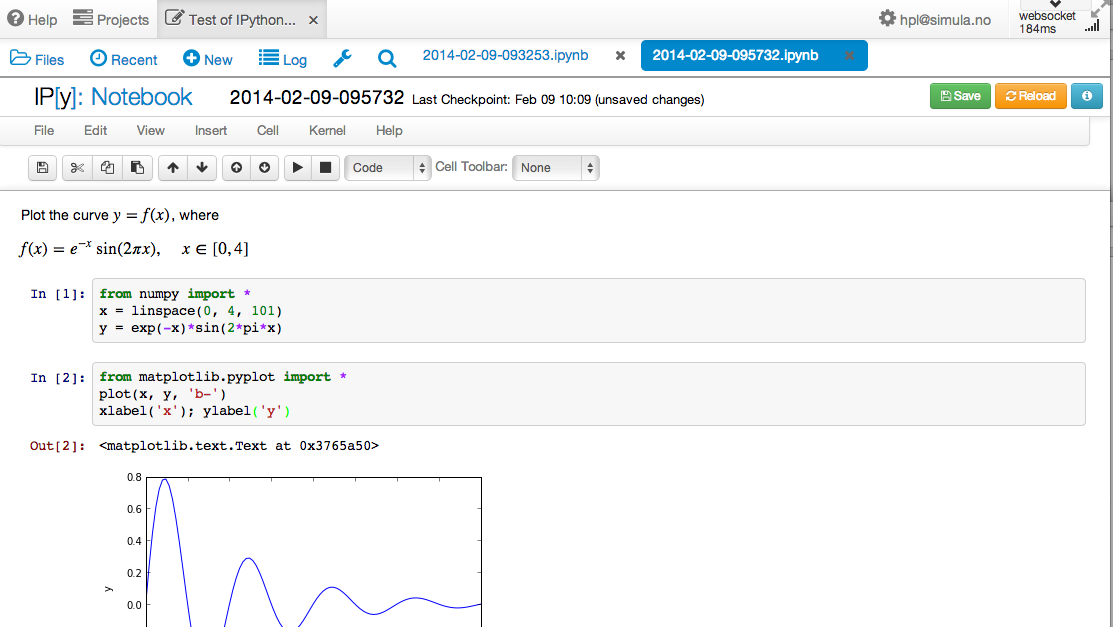$$\newcommand{\Oof}{\mathcal{O}(#1)} \newcommand{\F}{\boldsymbol{F}} \newcommand{\J}{\boldsymbol{J}} \newcommand{\x}{\boldsymbol{x}} \renewcommand{\c}{\boldsymbol{c}}$$

# Writing IPython notebooks

The IPython notebook is a splendid interactive tool for doing science, but it can also be used as a platform for developing Python code. You can either run it locally on your computer or in a web service like SageMathCloud or Wakari. Installation on your computer is trivial on Ubuntu, just sudo apt-get install ipython-notebook, and also on Windows and Mac by using Anaconda or Enthought Canopy for the Python installation.

The interface to the notebook is a web browser: you write all the code and see all the results in the browser window. There are excellent YouTube videos on how to use the IPython notebook, so here we provide a very quick "step zero" to get anyone started.

## A simple program in the notebook

Start the IPython notebook locally by the command ipython notebook or go to SageMathCloud or Wakari as described above. The default input area is a cell for Python code. Type

g = 9.81
v0 = 5
t = 0.6
y = v0*t - 0.5*g*t**2


in a cell and run the cell by clicking on Run Selected (notebook running locally on your machine) or on the "play" button (notebook running in the cloud). This action will execute the Python code and initialize the variables g, v0, t, and y. You can then write print y in a new cell, execute that cell, and see the output of this statement in the browser. It is easy to go back to a cell, edit the code, and re-execute it.

To download the notebook to your computer, choose the File - Download as menu and select the type of file to be downloaded: the original notebook format (.ipynb file extension) or a plain Python program version of the notebook (.py file extension).

## Mixing text, mathematics, code, and graphics

The real strength of IPython notebooks arises when you want to write a report to document how a problem can be explored and solved. As a teaser, open a new notebook, click in the first cell, and choose Markdown as format (notebook running locally) or switch from Code to Markdown in the pull-down menu (notebook in the cloud). The cell is now a text field where you can write text with Markdown syntax. Mathematics can be entered as LaTeX code. Try some text with inline mathematics and an equation on a separate line:

Plot the curve $y=f(x)$, where

$$f(x) = e^{-x}\sin (2\pi x),\quad x\in [0, 4]$$


Execute the cell and you will see nicely typeset mathematics in the browser. In the new cell, add some code to plot $$f(x)$$:

import numpy as np
import matplotlib.pyplot as plt
%matplotlib inline  # make plots inline in the notebook

x = np.linspace(0, 4, 101)
y = np.exp(-x)*np.sin(2*pi*x)
plt.plot(x, y, 'b-')
plt.xlabel('x'); plt.ylabel('y')


Executing these statements results in a plot in the browser, see Figure 65. It was popular to start the notebook by ipython notebook --pylab to import everything from numpy and matplotlib.pyplot and make all plots inline, but the --pylab option is now officially discouraged. If you want the notebook to behave more as MATLAB and not use the np and plt prefix, you can instead of the first three lines above write %pylab.

Figure 65: Example on an IPython notebook.